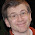## Tuesday, June 3, 2014

### How-to Create Math Documents Quick and Easy with g(math)

Math teachers... have you ever tried to make a custom worksheet for your class and about 45 min later you want to chuck your computer out the window?  Let's face it, trying to create math equations in a word processor sucks!

That's where teacher John McGowan comes to the rescue with his new Google add-on called g(math).

This cool little equation editor that allows you to bust out math equations faster than Google Drive's built-in equation editor.

To get you up and going, I'm going to provide a written and video tutorial to guide you through the process.

## Written Guide: Part 2 - Adding a Graph

### Step 2: Type in your equation into the field provided.  You can also put in a second equation if you have a system of equations.

Note: If you need help with what to type to make a multiplication sign or any other math operator, then you can go here.

### Step 3: Voila! There's your beautiful graph!

#### 1 comment:

1.Can I create randomized quizzes where students have to solve 2x+3y=12 for x, but the 2, the 3 and 12 might be different for every student?